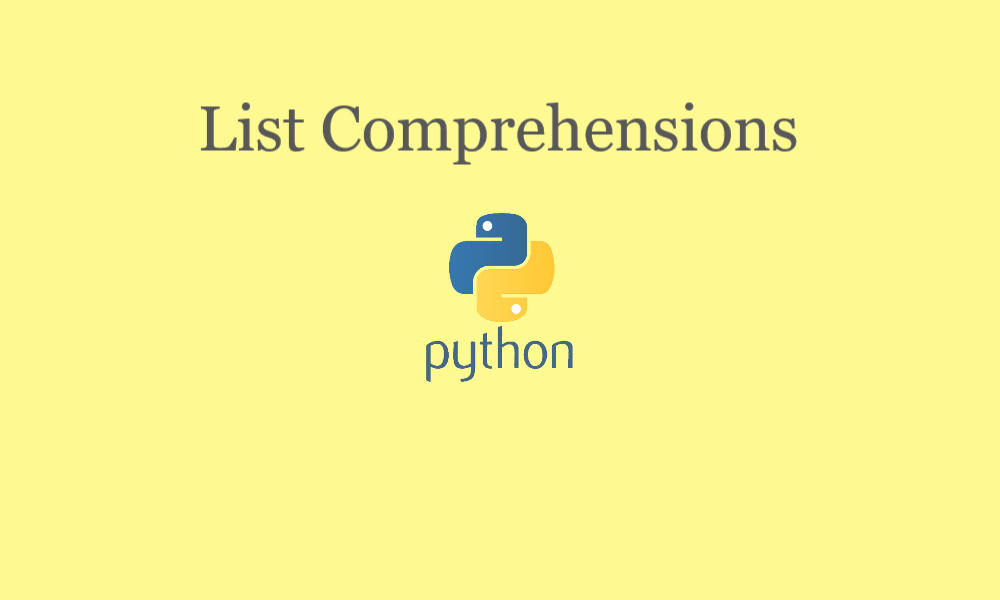# SURPRISE BUT LIST COMPREHENSION IS 10X FASTER THAN LOOPS IN PYTHON – 9## OVERVIEW

In this blog, you will learn,

• What is list comprehension?
• Syntax and it’s implementation
• How does list comprehension works in python?
• List comprehension is 10x faster than loops

## What is List Comprehension?

List comprehension is the elegant and the faster way to create a new list with the shorter syntax . In list comprehension we use for loop in such a way that it is written in just one line of code, that’s why it is also know as the in-line for loop. Output of the list comprehension is a new list with the items that are generated based on the for loop and/or with the conditions applied to it. Below is the syntax for the list comprehension,

```Syntax:
[<expression> for <var-name> in <iterator>]```

## WORKING OF LIST COMPREHENSION

In list comprehension, firstly we’ll write the code as per the above syntax. Here, a for loop is created just like a normal way to access the value on every iteration except for the expression, here the expression is returned in the same line of code before the for loop is written. Now let’s understand it in more details with an example.

Now our task is to create a list with the square of all the first 10 natural numbers with in-line for loop.

``````output=[i**2 for i in range(1,11)]
print(output)``````
```Output:
[1, 4, 9, 16, 25, 36, 49, 64, 81, 100]```

In the above example, you can see how easy it was to write a program to create a list containing he square of all the first 10 natural numbers. So, from the above example you can understand i**2 is the expression to return the value on every iteration with respect to the iterator(which is range function in our case with values from 1 to 10).

Now let’s take another example of list comprehension with the conditional statement. Now our task is to return a list containing the square of all the first 10 even natural numbers.

``````output=[i**2 for i in range(1,20) if i%2==0]
print(output)``````
`Output:        [4, 16, 36, 64, 100, 144, 196, 256, 324]`

So, with the above example you must have understood that how we can add the conditional statement to the list comprehension and how the result is generated on the basis of condition. In the above example you can see that we have put a condition to check that a number is even or not with in the range from 1 to 20. If the number is even then the square of that number is returned to the list otherwise, nothing is done.

## List comprehension is 10x faster than loops

Now let’s take and example to prove that the in-line for executes almost 10 times faster than the normal loop. Below is the code to prove that,

`import time## In-line for loopt1=time.time()output=[i**3 if i%2==0 else i**4 for i in range(1,20000) ]time_list=time.time()-t1print("Time taken for in-line for loop:",time_list,'seconds')t2=time.time()out=[]for i in range(1,20000):    if i % 2 == 0:        out.append(i**3)    else:        out.append(i**4)time_for=time.time()-t2print("Time taken for normal for loop :",time_for,"seconds")`

Time to wrap up now. Hope you liked our content on How and why to use list comprehension in python. See you in our next blog, thanks for reading our blog and do leave a comment below to help us improve the content to serve you all of our experience with you. Stay tuned with us for more python contents.

Also check out our other playlist Rasa Chatbot, Internet of things, Docker, Python Programming, etc.
Become a member of our social family on youtube here.

Stay tuned and Happy Learning.

Ashish Saini

I am a software developer for Python/Machine Learning. Also, I’m Rasa Hero at Rasa. Also, I’m a youtuber where I regulary uploads the videos to help this generation learn about the technology just like they are playing games.